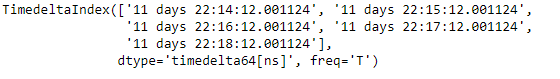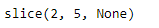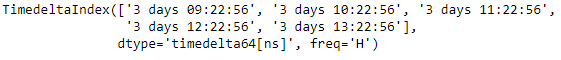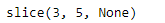Related Articles
Python | Pandas TimedeltaIndex.slice_indexer
• Last Updated : 06 Jan, 2019

Python is a great language for doing data analysis, primarily because of the fantastic ecosystem of data-centric python packages. Pandas is one of those packages and makes importing and analyzing data much easier.

Pandas` TimedeltaIndex.slice_indexer()` function compute the slice indexer for input labels and step for an ordered TimedeltaIndex object. The function assumes that the data is sorted.

Syntax : TimedeltaIndex.slice_indexer(start=None, end=None, step=None, kind=None)

Parameters :
start : If None, defaults to the beginning
end : If None, defaults to the end
step : int, default None
kind : string, default None

Return : indexer : ndarray or slice

Example #1: Use `TimedeltaIndex.slice_indexer()` function to compute the slice indexer for the passed label in the given TimedeltaIndex object.

 `# importing pandas as pd``import` `pandas as pd`` ` `# Create the TimedeltaIndex object``tidx ``=` `pd.TimedeltaIndex(start ``=``'11 days 22:14:12.001124'``,``                                   ``periods ``=` `5``, freq ``=``'T'``)`` ` `# Print the TimedeltaIndex object``print``(tidx)`

Output :Now we will use the `TimedeltaIndex.slice_indexer()` function to find the slice value for the passed labels.

 `# find the slice indexer``tidx.slice_indexer(``'11 days 22:15:20.001124'``)`

Output :As we can see in the output, the `TimedeltaIndex.slice_indexer()` function has returned the position as well as the number of elements in the tidx object.

Example #2: Use `TimedeltaIndex.slice_indexer()` function to compute the slice indexer for the passed label in the given TimedeltaIndex object.

 `# importing pandas as pd``import` `pandas as pd`` ` `# Create the TimedeltaIndex object``tidx ``=` `pd.TimedeltaIndex(start ``=``'03 days 09:22:56'``,``                            ``periods ``=` `5``, freq ``=``'H'``)`` ` `# Print the TimedeltaIndex object``print``(tidx)`

Output :Now we will use the `TimedeltaIndex.slice_indexer()` function to find the slice value for the passed labels.

 `# find the slice indexer``tidx.slice_indexer(``'3 days 12:20:56'``)`

Output :As we can see in the output, the `TimedeltaIndex.slice_indexer()` function has returned the position as well as the number of elements in the tidx object.

Attention geek! Strengthen your foundations with the Python Programming Foundation Course and learn the basics.

To begin with, your interview preparations Enhance your Data Structures concepts with the Python DS Course.

My Personal Notes arrow_drop_up